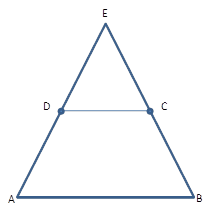Chapter 4.4, Problem 17EElementary Geometry For College St...

7th Edition
Alexander + 2 others
ISBN: 9781337614085

Solutions

Chapter
SectionElementary Geometry For College St...

7th Edition
Alexander + 2 others
ISBN: 9781337614085
Textbook Problem

Given: ABCD is an isosceles trapezoid.Prove: Δ A B E is isosceles.Exercises 17, 18

To determine

To Prove:

The triangle is isosceles.

Explanation

Consider the following diagram.

Given: ABCD is an isosceles trapezoid.

To Prove: ΔABE is isosceles.

Plan: Draw auxiliary altitudes DF and CG, in such a way that DFAB and CGAB.

We know that two parallel line are everywhere equidistant

Still sussing out bartleby?

Check out a sample textbook solution.

See a sample solution

The Solution to Your Study Problems

Bartleby provides explanations to thousands of textbook problems written by our experts, many with advanced degrees!

Get Started

Solve the equations in Exercises 112 for x (mentally, if possible). x+1=0

Finite Mathematics and Applied Calculus (MindTap Course List)

Define the terms population and sample, and explain the role of each in a research study.

Essentials of Statistics for The Behavioral Sciences (MindTap Course List)

True or False: f(x)=x2(x+1)x is continuous for all real numbers.

Study Guide for Stewart's Single Variable Calculus: Early Transcendentals, 8th

A parameterization of the curve at the right is:

Study Guide for Stewart's Multivariable Calculus, 8th